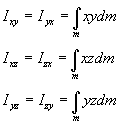Calculating angular momentum for a rigid body

Alternate forms for calculating angular momentum: A useful expression for calculating the angular momentum is based on introducing a point A fixed to the body and using the kinematic relations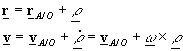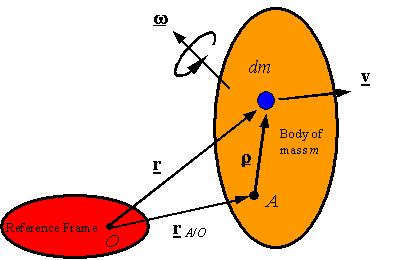where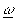is the angular velocity of the body as seen by an observer on the reference frame. Introducing these relations into the expression for the angular velocity yields the expression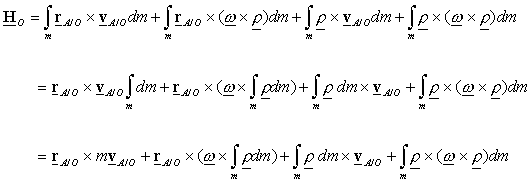Angular momentum of a body rotating around a fixed point on the reference frame: If the body is pinned or ball and socket connected to the reference frame at O, then one can simplify the above expressions by taking A equal to O to get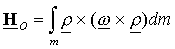Using an arbitrary intermediate point A on the rigid body: If A is an arbitrary point on the rigid body, one can use the relation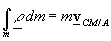to get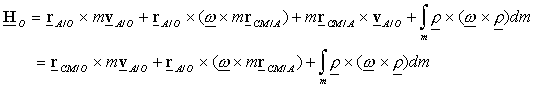Using the intermediate point A as the center of mass: If one sets A as the center of mass, then the expression for the angular momentum about O becomesSince the mass of the body is “evenly” distributed around the center of mass, one can conclude that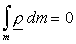Therefore, one obtains the expression for angular momentum about an arbitrary point as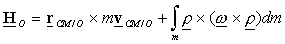Note: in all the above expressions for angular momentum an integral needs to be calculated of the form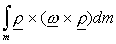A general expression for this can be found by selecting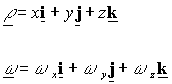and directly calculating the integral to get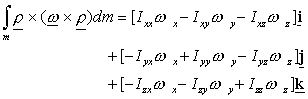where the moments of inertia are given byand the products of inertia are given by